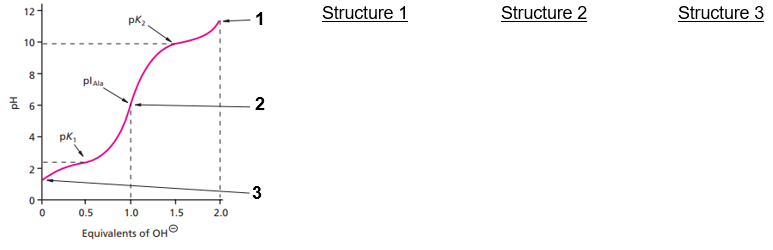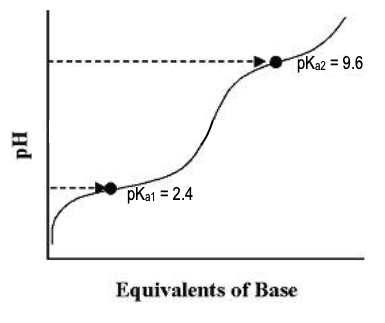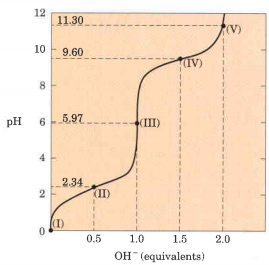Clutch Prep is now a part of Pearson
Ch. 3 - Amino AcidsWorksheetSee all chapters

# Titrations of Amino Acids with Non-Ionizable R-Groups

See all sections
Sections
Amino Acid Groups
Amino Acid Three Letter Code
Amino Acid One Letter Code
Amino Acid Configuration
Essential Amino Acids
Nonpolar Amino Acids
Aromatic Amino Acids
Polar Amino Acids
Charged Amino Acids
How to Memorize Amino Acids
Zwitterion
Non-Ionizable Vs. Ionizable R-Groups
Isoelectric Point
Isoelectric Point of Amino Acids with Ionizable R-Groups
Titrations of Amino Acids with Non-Ionizable R-Groups
Titrations of Amino Acids with Ionizable R-Groups
Amino Acids and Henderson-Hasselbalch

Concept #1: Titration Curve Review

Concept #2: Titrations Of Amino Acids With Non-Ionizable R-Groups

Practice: Draw the predominate structures of Leu at each of the indicated sections ( 1, 2, 3) on its titration curve.Practice: Calculate the pI of Ile using its titration curve. Mark the approximate position of the pI on the titration curve.Practice: Which of the following compounds would make for the best buffer at pH 8?

Practice: Identify the region(s) on glycine’s titration (I, II, III, IV, or V) that corresponds with each statement below:a. Region where Gly predominant species has net charge of +1. ________

b. Region where the average net charge of Gly is +½. ________

c. Region where ½ of Gly’s amino groups are ionized. ________

d. Region where the pH = pKa of carboxyl group. ________

e. Region where the pH = pKa of amino group. ________

f. Regions where Gly has its maximum buffering capacity. _____________

g. Region where the average net charge of Gly is 0. ________

h. Region where Gly’s carboxyl group has been completely titrated. ________

i. Region where Gly has been completely titrated. ________

j. Region where Gly’s predominant species is a zwitterion. ________

k. Region where the average net charge of Gly is -1. ________

l. Region where Gly is a 50:50 mixture of protonated & deprotonated carboxyl group. ________

m. Region indicating Gly’s isoelectric point (pI). ________

n. Region indicating the end of Gly’s titration. ________

o. Regions where Gly has poor buffering power. ____________________